## Process-Scheduling

 Question 1

Consider the following four processes with arrival times (in milliseconds) and their length of CPU bursts (in milliseconds) as shown below: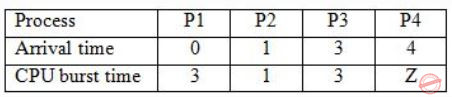These processes are run on a single processor using preemptive Shortest Remaining Time First scheduling algorithm. If the average waiting time of the processes is 1 millisecond, then the value of Z is _____.

 A 2 B 7 C 1 D 4
Operating-Systems       Process-Scheduling       GATE 2019       Video-Explanation
Question 1 Explanation:
This is the Gantt chart till time = 4 unitsAt this point P4 arrives with burst 'Z' & P3 is in queue with burst 3.
P1 & P2 have executed with P1 incurred delay 1unit & P2 0units.
Hence, Avg = 0+1+x/4 =1
⇒ x=3, the next delay should be 3. It would happen if assume Z=2.
It executes and completes at 6.
P3 will wait totally for 3units.
Hence, Avg=1.Z=2
 Question 2

Consider the following CPU processes with arrival times (in milliseconds) and length of CPU bursts (in milliseconds) as given below: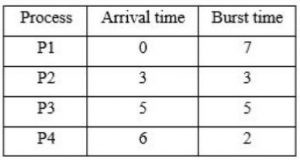If the pre-emptive shortest remaining time first scheduling algorithm is used to schedule the processes, then the average waiting time across all processes is __________ milliseconds.

 A 3 B 4 C 5 D 6
Operating-Systems       Process-Scheduling       GATE 2017 [Set-1]       Video-Explanation
Question 2 Explanation:Question 3

Consider the set of processes with arrival time (in milliseconds), CPU burst time (in milliseconds), and priority (0 is the highest priority) shown below. None of the processes have I/O burst time.The average waiting time (in milliseconds) of all the processes using preemptive priority scheduling algorithm is __________.

 A 29 B 30 C 31 D 32
Operating-Systems       Process-Scheduling       GATE 2017 [Set-2]       Video-Explanation
Question 3 Explanation: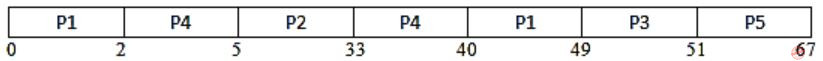Waiting Time = 0 + (33 - 5) + (40 - 2) + (49 - 12) + (51 - 9) = 145
Average waiting time: 145/5 = 29
 Question 4

Consider an arbitrary set of CPU-bound processes with unequal CPU burst lengths submitted at the same time to a computer system. Which one of the following process scheduling algorithms would minimize the average waiting time in the ready queue?

 A Shortest remaining time ﬁrst B Round-robin with time quantum less than the shortest CPU burst C Uniform random D Highest priority ﬁrst with priority proportional to CPU burst length
Operating-Systems       Process-Scheduling       GATE 2016 [Set-1]       Video-Explanation
Question 4 Explanation:
From the above question, we can get the following information.
We can consider an arbitrary set of CPU-bound processes with unequal CPU burst lengths submitted at the same time to a computer system.
We have to choose the appropriate process scheduling algorithms, which would minimize the average waiting time in the ready queue.
Waiting time is the time for which process is ready to run but not executed by CPU scheduler.
In all CPU Scheduling algorithms, shortest job first is optimal.
It gives minimum turnaround time, minimum average waiting time and high throughput and the most important thing is that shortest remaining time first is the preemptive version of shortest job first.
This scheduling algorithm may lead to starvation because if the short processes are added to the CPU scheduler continuously then the currently running process will never be able to execute as they will get pre-empted but here all the processes are arrived at same time so there will be no issue such as starvation.
SRTF would be same as SJF.
So, A is the answer. Shortest remaining time first.
 Question 5

Consider the following processes, with the arrival time and the length of the CPU burst given in milliseconds. The scheduling algorithm used is preemptive shortest remaining-time ﬁrst.The average turn around time of these processes is _________ milliseconds.

 A 8.25 B 8.26 C 8.27 D 8.28
Operating-Systems       Process-Scheduling       GATE 2016 [Set-2]       Video-Explanation
Question 5 Explanation:
Here the scheduling algorithm used is preemptive shortest remaining-time first.
To answer the question we need to design the gantt chart: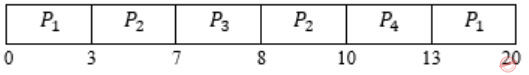In this algorithm, the processes will be scheduled on the CPU which will be having least remaining burst time.
Turnaround Time (TAT) = Completion Time (CT) - Arrival Time (AT)
TAT for P1 = 20 - 0 = 20,
TAT for P2 = 10 - 3 = 7,
TAT for P3 = 8 - 7 = 1,
TAT for P4 = 13 - 8 = 5.
Total TAT = 20 + 7 + 1 + 5 = 33 / 4 = 8.25 (Avg. TAT)
 Question 6

Consider a uniprocessor system executing three tasks T1, T2 and T3, each of which is composed of an infinite sequence of jobs (or instances) which arrive periodically at intervals of 3, 7 and 20 milliseconds, respectively. The priority of each task is the inverse of its period and the available tasks are scheduled in order of priority, with the highest priority task scheduled first. Each instance of T1, T2 and T3 requires an execution time of 1, 2 and 4 milliseconds, respectively. Given that all tasks initially arrive at the beginning of the 1st millisecond and task preemptions are allowed, the first instance of T3 completes its execution at the end of ______________ milliseconds.

 A 13 B 12 C 15 D 16
Operating-Systems       Process-Scheduling       GATE 2015 [Set-1]
Question 6 Explanation:
All the processes or tasks are available at the begining of 1st millisecond, means at t=0. So, Gantt chart will be as follows:∴ At the end of 12 milliseconds, 1st instance of T3 will complete its execution.
 Question 7

The maximum number of processes that can be in Ready state for a computer system with n CPUs is

 A n B n2 C 2n D Independent of n
Operating-Systems       Process-Scheduling       GATE 2015 [Set-3]
Question 7 Explanation:
Number of processes which are in running processes will be atmost n as there are n processors.
Maximum number of processes that will be in ready state is independent of number of processors.
 Question 8

For the processes listed in the following table, which of the following scheduling schemes will give the lowest average turnaround time?A First Come First Serve B Non-preemptive Shortest Job First C Shortest Remaining Time D Round Robin with Quantum value two
Operating-Systems       Process-Scheduling       GATE 2015 [Set-3]
Question 8 Explanation:FCFS:TAT for A = completion time(A) - AT(A) = 3 - 0 = 3
TAT of B = 9 - 1 = 8
TAT of C = 13 - 4 = 9
TAT of D = 15 - 6 = 9
∴ Avg. TAT = 29/4
SJF: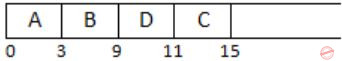TAT of A = 3 - 0 = 3
TAT of B = 9 - 1 = 8
TAT of C = 15 - 4 = 11
TAT of D = 11 - 6 = 5
∴ Avg. TAT = 27/4
SRTF:TAT of A = 3 - 0 = 3
TAT of B = 15 - 1 = 14
TAT of C = 8 - 4 = 4
TAT of D = 10 - 6 = 4
∴ Avg. TAT = 25/4
RR:TAT of A = 5 - 0 = 5
TAT of B = 15 - 1 = 14
TAT of C = 13 - 4 = 9
TAT of D = 11 - 6 = 5
∴ Avg. TAT = 33/4
∴ SRTF has lowest Avg. TAT.
 Question 9

Consider the following set of processes that need to be scheduled on a single CPU. All the times are given in milliseconds.Using the shortest remaining time first scheduling algorithm, the average process turnaround time (in msec) is ________.

 A 7.2 B 7.3 C 7.4 D 7.5
Operating-Systems       Process-Scheduling       GATE 2014 [Set-1]
Question 9 Explanation:
Using SRTF:TAT(A) = 8-0 = 8, TAT(B)= 5-3=2, TAT(C)= 12-5=7, TAT(D)= 21-7= 14, TAT(E)=15-10=5
Average turnaround time = 8+2+7+14+5/ 5 = 7.2ms
 Question 10

Three processes A, B and C each execute a loop of 100 iterations. In each iteration of the loop, a process performs a single computation that requires tc CPU milliseconds and then initiates a single I/O operation that lasts for tio milliseconds. It is assumed that the computer where the processes execute has sufficient number of I/O devices and the OS of the computer assigns different I/O devices to each process. Also, the scheduling overhead of the OS is negligible. The processes have the following characteristics:

``` Process id      tc      tio
A        100 ms    500 ms
B        350 ms    500 ms
C        200 ms    500 ms```

The processes A, B, and C are started at times 0, 5 and 10 milliseconds respectively, in a pure time sharing system (round robin scheduling) that uses a time slice of 50 milliseconds. The time in milliseconds at which process C would complete its first I/O operation is ___________.

 A 1000 B 1001 C 1002 D 1003
Operating-Systems       Process-Scheduling       GATE 2014 [Set-2]
Question 10 Explanation:
Gantt chart is shown below:So 'C' completes its I/O at 1000 time units.
 Question 11

Consider the 3 processes, P1, P2 and P3 shown in the table.

```Process           Arrival time         Time Units Required
P1                0                         5
P2                1                         7
P3                3                         4```

The completion order of the 3 processes under the policies FCFS and RR2 (round robin scheduling with CPU quantum of 2 time units) are

 A FCFS: P1, P2, P3 RR2: P1, P2, P3 B FCFS: P1, P3, P2 RR2: P1, P3, P2 C FCFS: P1, P2, P3 RR2: P1, P3, P2 D FCFS: P1, P3, P2 RR2: P1, P2, P3
Operating-Systems       Process-Scheduling       GATE 2012
Question 11 Explanation:
FCFS is - P1, P2, P3
FCFS is clear.
RR Queue: In RR queue time slot is of 2 units.
Processes are assigned in following order
P1, P2, P1, P3, P2, P1, P3, P2, P2
This question used the ready queue concept. At t=2, P2 starts and P1 is sent to the ready queue and at t=3 P3 arrives so then the job P3 is queued in the ready queue after P1. So at t=4, again P1 is executed then P3 is executed for the first time at t=6.
RR2: P1, P3, P2
So option C.
 Question 12

Consider the following table of arrival time and burst time for three processes P0, P1 and P2.The pre-emptive shortest job first scheduling algorithm is used. Scheduling is carried out only at arrival or completion of processes. What is the average waiting time for the three processes?

 A 5.0 ms B 4.33 ms C 6.33 ms D 7.33 ms
Operating-Systems       Process-Scheduling       GATE 2011
Question 12 Explanation:CT = Completion time
TAT = Turn Around Time
WT = Waiting Time
TAT = CT - AT
WT = TAT - BT
Gantt Chart using pre-emptive shortest job first scheduling algorithm,Avg. WT = 4+0+11/3 = 5ns
 Question 13

Which of the following statements are true?

I. Shortest remaining time first scheduling may cause starvation
II. Preemptive scheduling may cause starvation
III. Round robin is better than FCFS in terms of response time
 A I only B I and III only C II and III only D I, II and III
Operating-Systems       Process-Scheduling       GATE 2010
Question 13 Explanation:
- In SRTF longer bursts will suffer from starvation.
- Pre-emptive scheduling causes starvation (for example lower priority jobs are waiting).
- Best Response time is given by RR.
 Question 14

In the following process state transition diagram for a uniprocessor system, assume that there are always some processes in the ready state:Now consider the following statements:

I. If a process makes a transition D, it would result in another process making transition A immediately.
II. A process P2 in blocked state can make transition E while another process P1 is in running state.
III. The OS uses preemptive scheduling.
IV. The OS uses non-preemptive scheduling.

Which of the above statements are TRUE?

 A I and II B I and III C II and III D II and IV
Operating-Systems       Process-Scheduling       GATE 2009
Question 14 Explanation:
Statement I is false, if a process makes a transition D, then it result to perform a transition B immediately not A.
Statement II is true, a process can move to ready state when I/O completes irrespective of other process being in running state or not.
Statement III is true, the transition C, represents preemptive scheduling.
Statement IV is false, because it is preemptive scheduling.
Correct Answer is Option-C (II & III are true).
 Question 15

An operating system uses Shortest Remaining Time first (SRT) process scheduling algorithm. Consider the arrival times and execution times for the following processes:

```
Process     Execution time     Arrival time
P1             20                0
P2             25                15
P3             10                30
P4             15                45
```

What is the total waiting time for process P2?

 A 5 B 15 C 40 D 55
Operating-Systems       Process-Scheduling       GATE 2007
Question 15 Explanation:Waiting time for process P2 = TAT - Execution time
= Completion time - AT - ET
= 55 - 15 - 25
= 15
 Question 16

Consider three CPU-intensive processes, which require 10, 20 and 30 time units and arrive at times 0, 2 and 6, respectively. How many context switches are needed if the operating system implements a shortest remaining time first scheduling algorithm? Do not count the context switches at time zero and at the end.

 A 1 B 2 C 3 D 4
Operating-Systems       Process-Scheduling       GATE 2006
Question 16 Explanation:Total no.of context switches is 2.
 Question 17

Consider three processes (process id 0, 1, 2 respectively) with compute time bursts 2, 4 and 8 time units. All processes arrive at time zero. Consider the longest remaining time first (LRTF) scheduling algorithm. In LRTF ties are broken by giving priority to the process with the lowest process id. The average turnaround time is:

 A 13 units B 14 units C 15 units D 16 units
Operating-Systems       Process-Scheduling       GATE 2006
Question 17 Explanation:
Algorithm: LRTF (Longest Remaining Time First)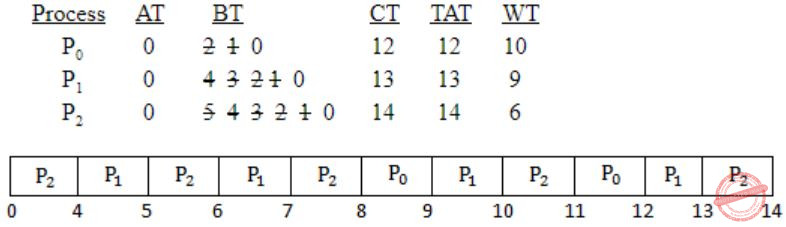Avg TAT = 12+13+14/3 = 39/3 = 13 units
 Question 18

Consider three processes, all arriving at time zero, with total execution time of 10, 20 and 30 units, respectively. Each process spends the first 20% of execution time doing I/O, the next 70% of time doing computation, and the last 10% of time doing I/O again. The operating system uses a shortest remaining compute time first scheduling algorithm and schedules a new process either when the running process gets blocked on I/O or when the running process finishes its compute burst. Assume that all I/O operations can be overlapped as much as possible. For what percentage of time does the CPU remain idle?

 A 0% B 10.6% C 30.0% D 89.4%
Operating-Systems       Process-Scheduling       GATE 2006
Question 18 Explanation:Total time needed to complete the execution = 47
Idle time = 2+3 = 5
Percentage of Idle time = 5/47 × 100 =10.6%
 Question 19

Consider the following set of processes, with the arrival times and the CPU-burst times given in milliseconds.

```  Process   Arrival Time    Burst Time
P1          0              5
P2          1              3
P3          2              3
P4          4              1 ```

What is the average turnaround time for these processes with the preemptive shortest remaining processing time first (SRPT) algorithm?

 A 5.5 B 5.75 C 6 D 6.25
Operating-Systems       Process-Scheduling       GATE 2004
Question 19 Explanation:
Uses SRPT Algorithm:Avg. TAT = 12+3+6+1/4 = 22/4 = 5.50
 Question 20

A uni-processor computer system only has two processes, both of which alternate 10ms CPU bursts with 90ms I/O bursts. Both the processes were created at nearly the same time. The I/O of both processes can proceed in parallel. Which of the following scheduling strategies will result in the least CPU utilization (over a long period of time) for this system?

 A First come first served scheduling B Shortest remaining time first scheduling C Static priority scheduling with different priorities for the two processes D Round robin scheduling with a time quantum of 5 ms
Operating-Systems       Process-Scheduling       GATE 2003
Question 20 Explanation:
We have two processes P and Q. These process have 10ms CPU burst time and 90ms I/O bursts.
(i) FCFS:
0-10: Process 1 can execute
10-20: Process 2 can execute
100-110: Process 1 Terminate
110-120: Process 2 Terminate
CPU utilization = 20/100 [In every 100ms it utilizes]
=20%
(ii) SRTF: can process P and Q same as FCFS
then CPU utilization = 20%
(iii) Round robin: with TQ-5
0-5: Process P1
5-10: Process P2
10-15: Process P1
15-20: Process P2
105-110: Process P1
110-115: Process P2
CPU utilization = 20/105 = 19.5
 Question 21

Which of the following scheduling algorithms is non-preemptive?

 A Round Robin B First-In First-Out C Multilevel Queue Scheduling D Multilevel Queue Scheduling with Feedback
Operating-Systems       Process-Scheduling       GATE 2002
Question 21 Explanation:
First-in first-out scheduling algorithm is non-preemptive. In this whichever the process enter into ready queue first that will be served first.
 Question 22

Consider a set of n tasks with known runtimes r1, r2, ..., rn to be run on a uniprocessor machine. Which of the following processor scheduling algorithms will result in the maximum throughput?

 A Round-Robin B Shortest-Job-First C Highest-Response-Ratio-Next D First-Come-First-Served
Operating-Systems       Process-Scheduling       GATE 2001
Question 22 Explanation:
First we know what is throughput. It means total number of tasks executed per unit time. SJF executes shortest job very early so throughput increases in the case of SJF.
 Question 23

Consider n processes sharing the CPU in a round-robin fashion. Assuming that each process switch takes s seconds, what must be the quantum size q such that the overhead resulting from process switching is minimized but at the same time each process is guaranteed to get its turn at the CPU at least every t seconds?

 A q ≤ t-ns/n-1 B q ≥ t-ns/n-1 C q ≤ t-ns/n+1 D q ≥ t-ns/n+1
Operating-Systems       Process-Scheduling       GATE 1998
Question 23 Explanation:Question 24

The process state transition diagram in below figure is representative ofA a batch operating system B an operating system with a preemptive scheduler C an operating system with a non-preemptive scheduler D a uni-programmed operating system
Operating-Systems       Process-Scheduling       GATE 1996
Question 24 Explanation:
Transaction from running → ready present.
So this is preemptive.
 Question 25

Four jobs to be executed on a single processor system arrive at time 0+ in the order A, B, C, D. their burst CPU time requirements are 4, 1, 8, 1 time units respectively. The completion time of A under round robin scheduling with time slice of one time unit is

 A 10 B 4 C 8 D 9
Operating-Systems       Process-Scheduling       GATE 1996
Question 25 Explanation:
Time quantum is 1 unit.∴ Completion time of A is 9 unit.
 Question 26

Consider the following statements about process state transitions for a system using preemptive scheduling.

I. A running process can move to ready state.
II. A ready process can move to running state.
III. A blocked process can move to running state.
IV. A blocked process can move to ready state.

Which of the above statements are TRUE?

 A II and III only B I, II and III only C I, II, III and IV D I, II and IV only
Operating-Systems       Process-Scheduling       GATE 2020
Question 26 Explanation:Question 27

Consider the following set of processes, assumed to have arrived at time 0. Consider the CPU scheduling algorithms Shortest Job First (SJF) and Round Robin (RR). For RR, assume that the processes are scheduled in the order P1,P2,P3,P4.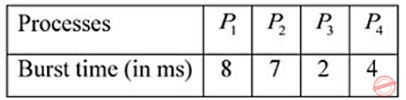If the time quantum for RR is 4 ms, then the absolute value of the difference between the average turnaround times (in ms) of SJF and RR (round off to 2 decimal places) is _____.

 A 5.25
Operating-Systems       Process-Scheduling       GATE 2020
Question 27 Explanation:
SJF:Turn Around Time = (21 – 0) + (13 – 0) + (2 – 0) + (6 – 0), Average = 42/4 = 10.50Turn Around Time (TAT) = (18 – 0) + (21 – 0) + (10 – 0) + (14 – 0), Average = 63/4 = 15.75
Absolute difference = |10.50-15.75| = 5.25
 Question 28

Let a memory have four free blocks of sizes 4k, 8k, 20k, 2k. These blocks are allocated following the best-fit strategy. The allocation requests are stored in a queue as shown below.The time at which the request for J7 will be completed will be

 A 16 B 19 C 20 D 37
Operating-Systems       Process-Scheduling       GATE 2007-IT
Question 28 Explanation:
At t = 0At t = 8At t = 10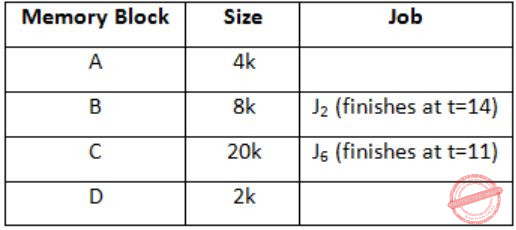At t = 11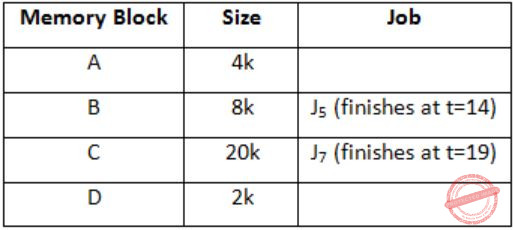J7 can be finished at t = 19.
 Question 29

Consider n jobs J1, J2,......Jn such that job Ji has execution time ti and a non-negative integer weight wi. The weighted mean completion time of the jobs is defined to be, where Ti is the completion time of job Ji. Assuming that there is only one processor available, in what order must the jobs be executed in order to minimize the weighted mean completion time of the jobs?

 A Non-decreasing order of ti B Non-increasing order of wi C Non-increasing order of witi D None-increasing order of wi/ti
Operating-Systems       Process-Scheduling       GATE 2007-IT
Question 29 Explanation:
Like OS concept, execute job which have more completion time in 1 sec i.e., find wi/ti for every process then arrange it in the decreasing order. So, we get complete time is minimum always.
So answer is the non-increasing order of wi/ti.
 Question 30

The process state transition diagram of an operating system is as given below.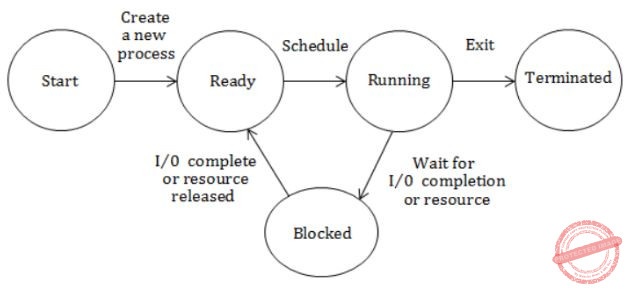Which of the following must be FALSE about the above operating system?

 A It is a multiprogrammed operating system B It uses preemptive scheduling C It uses non-preemptive scheduling D It is a multi-user operating system
Operating-Systems       Process-Scheduling       GATE 2006-IT
Question 30 Explanation:
Option B is wrong. Because given OS is non-preempting scheduling algorithm.
 Question 31

The arrival time, priority, and duration of the CPU and I/O bursts for each of three processes P1, P2 and P3 are given in the table below. Each process has a CPU burst followed by an I/O burst followed by another CPU burst. Assume that each process has its own I/O resource.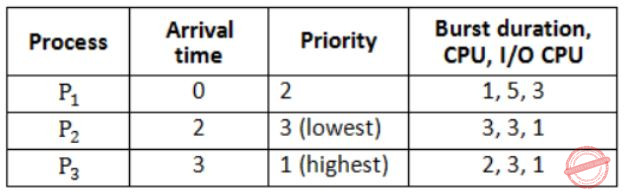The multi-programmed operating system uses preemptive priority scheduling. What are the finish times of the processes P1, P2 and P3 ?

 A 11, 15, 9 B 10, 15, 9 C 11, 16, 10 D 12, 17, 11
Operating-Systems       Process-Scheduling       GATE 2006-IT
Question 31 Explanation:
Gantt-chart:Hence, finish times of process.
P1 - 10
P2 - 11
P3 - 9
 Question 32
In real time operating systems, which of the following is the most suitable scheduling scheme?
 A Round Robin B First come first serve C preemptive D random scheduling
Operating-Systems       Process-Scheduling       Nielit Scientist-C 2016 march
Question 32 Explanation:
● A real-time operating system (RTOS) is any operating system (OS) intended to serve real-time applications that process data as it comes in, typically without buffer delays.
● Processing time requirements (including any OS delay) are measured in tenths of seconds or shorter increments of time.
● A real time system is a time bound system which has well defined fixed time constraints. Processing must be done within the defined constraints or the system will fail. They either are event driven or time sharing.
● Event driven systems switch between tasks based on their priorities while time sharing systems switch the task based on clock interrupts. Most RTOS’s use a pre-emptive scheduling algorithm.
 Question 33
In a lottery scheduler with 40 tickets, how we will distribute the tickets among 4 processes and such that each process gets 10%, 5%, 60% and 25% respectively?A a B b C c D d
Operating-Systems       Process-Scheduling       ISRO CS 2015
Question 33 Explanation:
Total number of tickets =40
Total 4 processors: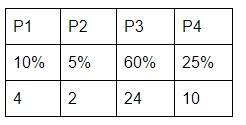Question 34

Which of the following statements is FALSE?

 A The long term scheduler controls the degree of multiprogramming B Multiple process of a single program cannot exist C Ready queue of the processes resides in main memory D A process can have multiple sub processes
Operating-Systems       Process-Scheduling       JT(IT) 2018 PART-B Computer Science
Question 34 Explanation:
→ Long term scheduler controls the degree of multiprogramming.
→ Ready queue of the processes resides in main memory.
→ A process can have multiple sub processes.
→ Multiple process of a single program cannot exist because each program can have only one process.
 Question 35
Consider three processes(process id 0,1,2 respectively) with compute time bursts 2,4 and 8 time units. All processes arrive at time zero. Consider the Longest remaining time first(LRTF) scheduling algorithm. In LRTF ties are broken by giving priority to the process with the lowest process id. The average turnaround time is:
 A 13 units B 14 units C 15 units D 16 units
Operating-Systems       Process-Scheduling       Nielit Scientist-B IT 22-07-2017
Question 35 Explanation:
Algorithm: LRTF (Longest Remaining Time First)Avg TAT = 12+13+14/3 = 39/3 = 13 units
 Question 36
Consider three processes, all arriving at time zero, with total execution time of 10,20 and 30 units, respectively. Each process spends the first 20% of execution time doing I/O, the next 70% of time doing computations, and the last 10% of time doing I/O again. The operating system uses a shortest remaining compute time first scheduling algorithm and schedules a new process either when the running process gets blocked on I/O or when the running process finishes its compute burst. Assume that all I/O operations can be overlapped as much as possible. For what percentage f time does the CPU remain idle?
 A 0% B 10.6% C 30.0% D 89.4%
Operating-Systems       Process-Scheduling       Nielit Scientist-B IT 22-07-2017
Question 36 Explanation:Total time needed to complete the execution = 47
Idle time = 2+3 = 5
Percentage of Idle time = 5/47 × 100 =10.6%
 Question 37
Consider three CPU-intensive processes which require 10,20 and 30 time units and arrive at times 0,2 and 6, respectively. How arrive at times 0,2 and 6, respectively. How many context switches are needed if the operating system implements a shortest remaining time first scheduling algorithm? Do not count the context switches at time zero and at the end.
 A 1 B 2 C 3 D 4
Operating-Systems       Process-Scheduling       Nielit Scientist-B IT 22-07-2017
Question 37 Explanation: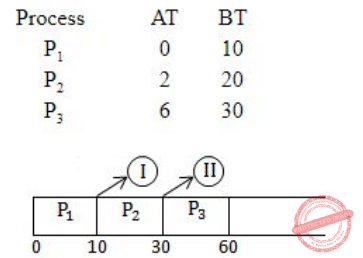Total no.of context switches is 2.
 Question 38
Which of the following process scheduling algorithm may lead to starvation?
 A FIFO B Round Robin C Shortest Job Next D None of the option
Operating-Systems       Process-Scheduling       Nielit Scientist-B IT 22-07-2017
Question 38 Explanation:
● Starvation is method in which process with high priorities continuously uses the resources preventing low priority process to acquire the resources
● In the Shortest job next scheduling shortest job process will run first and later higher burst time.Here the process with higher burst time need to wait for longer time.
 Question 39
A scheduling algorithm assigns priority proportional to the waiting time of a process. Every process starts with priority zero(the lowest priority). The scheduler re-evaluates the process priorities every T time units and decides the next process to schedule. Which one of the following is TRUE if the processes have no I/O operations and all arrive at time zero?
 A This algorithm is equivalent to the first come first serve algorithm B This algorithm is equivalent to the round-robin algorithm C This algorithm is equivalent t the shortest-job-first algorithm D This algorithm is equivalent to the shortest-remaining time-first algorithm
Operating-Systems       Process-Scheduling       Nielit Scientist-B IT 22-07-2017
Question 39 Explanation:
Let's take an example:Consider scheduler schedule processes priority after S times units so order of execution will be P1 P2 P3 P4 P1 P2 P3 P4 (S< execution time of any process) which is exactly same as round robin so answer – B.
 Question 40
Which of the following scheduling algorithm could result in starvation?
 A Priority B Round Robin C FCFS D none of the above
Operating-Systems       Process-Scheduling       Nielit Scientific Assistance IT 15-10-2017
Question 40 Explanation:
Priority scheduling algorithm could result starvation. Starvation is nothing but indefinite blocking.
 Question 41

State whether TRUE or FALSE

(i) Shortest remaining time (SRT) algorithm is the preemptive version of the shortest job next(SJN) CPU scheduling algorithm
(ii) A “context switch” is the mechanism to store and restore the state or context of a CPU in PCB
 A (i) True, (ii) True B (i) False, (ii) False C (i) False, (ii) True D (i) True, (ii) False
Operating-Systems       Process-Scheduling       JT(IT) 2018 PART-B Computer Science
Question 41 Explanation:
TRUE: Shortest remaining time (SRT) algorithm is the preemptive version of the shortest job next(SJN) CPU scheduling algorithm.
TRUE: A “context switch” is the mechanism to store and restore the state or context of a CPU in PCB.
 Question 42
If a process is runnable but is temporarily stopped to let another process run, in which state is the process said to be?
 A Running B Ready C Interrupted D Blocked
Operating-Systems       Process-Scheduling       KVS DEC-2013
Question 42 Explanation:
1. New
A program which is going to be picked up by the OS into the main memory is called a new process.
Whenever a process is created, it directly enters in the ready state, in which, it waits for the CPU to be assigned. The OS picks the new processes from the secondary memory and put all of them in the main memory. The processes which are ready for the execution and reside in the main memory are called ready state processes.
3. Running
One of the processes from the ready state will be chosen by the OS depending upon the scheduling algorithm.
4. Block or wait
From the Running state, a process can make the transition to the block or wait state depending upon the scheduling algorithm or the intrinsic behavior of the process.
 Question 43
The diagram given below representsA Process creation B Process state C Queueing diagram D Communication model
Operating-Systems       Process-Scheduling       KVS DEC-2013
Question 43 Explanation:
The above diagram represents queueing diagram. Various queues are
→ All processes, upon entering into the system, are stored in the Job Queue.
→ Processes in the Ready state are placed in the Ready Queue.
→ Processes waiting for a device to become available are placed in Device Queues. There are unique device queues available for each I/O device.
 Question 44
Consider a set of n tasks with known runtimes R​1​ ,R2​ ,Rn​ , to be run uniprocessor machine. Which of the following processor scheduling algorithm will result in the maximum throughput?
 A Priority scheduling B Round robin C FCFS D SJF
Operating-Systems       Process-Scheduling       KVS DEC-2017
Question 44 Explanation:
→ In shortest job first scheduling algorithm, the processor selects the process with the smallest execution time to execute next.
→ SJF scheduling algorithm will result in the maximum throughput
 Question 45
The problem of indefinite blockage of low priority jobs in general priority scheduling algorithm can be solved using
 A Swapping B Dirty bit C Aging D Compaction
Operating-Systems       Process-Scheduling       KVS DEC-2017
Question 45 Explanation:
→ Ageing is a scheduling technique used to avoid starvation. Fixed priority scheduling is a scheduling discipline, in which tasks queued for utilizing a system resource are assigned a priority each.
→ A task with a high priority is allowed to access a specific system resource before a task with a lower priority is allowed to do the same.
→ A disadvantage of this approach is that tasks assigned with a lower priority may be starved when a large number of high priority tasks are queued. Aging is used to gradually increase the priority of a task, based on its waiting time in the ready queue.
 Question 46
If the time quantum size is 2 units of an there is only one job of 14 time unit in a ready queue, the round robin scheduling algorithm will cause___ connected switches.
 A 1 B 5 C 6 D 7
Operating-Systems       Process-Scheduling       KVS DEC-2017
Question 46 Explanation:
→ Actually they are given single job, so when we are using round robin algorithm it won’t perform any context switch because we are executing same job.
→ But according to their intention will cause total 6 switches. 0-2, 2-4, 4-6, 6-8, 8-10, 10-12, 12-14. We are initially started with 0. So, It will take 6 connected switches
Note: We thought they made this question wrong.
 Question 47
Three processes are scheduled for execution.
Arrival times and time units required for execution are given below: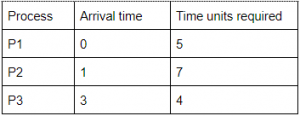Which of the following is the correct completion order if RR scheduling algorithm is used with time quantum of 2 units?
 A P1,P2,P3 B P1,P3,P2 C P3,P1,P2 D P2,P1,P3
Operating-Systems       Process-Scheduling       KVS 30-12-2018 Part B
Question 47 Explanation:
The gantt chart of the above RR scheduling algorithm isThe completion of order of processes are P1,P3,P2
 Question 48
Which of the following scheduling algorithms may cause starvation ?
a. First-come-first-served
b. Round Robin
c. Priority
d. Shortest process next
e. Shortest remaining time first
 A a, c and e B c, d and e C b, d and e D b, c and d
Operating-Systems       Process-Scheduling       UGC NET CS 2017 Jan -paper-2
Question 48 Explanation:
→ Starvation is the name given to the indefinite postponement of a process because it requires some resource before it can run, but the resource, though available for allocation, is never allocated to this process.
1. Priority: A process ready to run for CPU can wait indefinitely because of low priority
2. Shortest process next : longest process possibility to get starvation
3. Shortest remaining time first: longest process possibility to get starvation
Solution: Aging
→ FCFS and Round Robin scheduling never happen indefinite blocking.
 Question 49
The Unix Kernel maintains two key data structures related to processes, the process table and the user structure. Which of the following information is not the part of user structure?
 A File descriptor table B System call state C Scheduling parameters D Kernel stack
Operating-Systems       Process-Scheduling       UGC NET CS 2015 Jun- paper-2
Question 49 Explanation:
The Unix Kernel maintains two key data structures related to processes, the process table and the user structure.
1. File descriptor table
2. System call state
3. Kernel stack
Scheduling parameters: Process priority, amount of CPU time consumed recently, amount of time spent sleeping recently. Together, these are used to determine which process to run next.
 Question 50
Match the following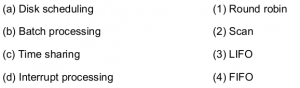A a-3, b-4, c-2, d-1 B a-4, b-3, c-2, d-1 C a-2, b-4, c-1, d-3 D a-3, b-4, c-1, d-2
Operating-Systems       Process-Scheduling       UGC NET CS 2004 Dec-Paper-2
Question 50 Explanation:
Disk scheduling→ SCAN,C-SCAN,LOOK,C-LOOK
Batch processing→ FIFO
Time sharing→ Round robin with the help of time quantum.
Interrupt processing→ LIFO
 Question 51
____________ is one of preemptive scheduling algorithm.
 A Shortest-Job-first B Round-robin C Priority based D Shortest-Job-next
Operating-Systems       Process-Scheduling       UGC NET CS 2006 Dec-paper-2
Question 51 Explanation:
→ SJF scheduling algorithm is either preemptive and Nonpreemptive.
→ Round robin is purely preemptive.
→ Priority based scheduling also preemptive and Nonpreemptive.
→ Shortest-Job-next scheduling algorithm is either preemptive and Nonpreemptive.
 Question 52
In processor management, round robin method essentially uses the preemptive version of __________ .
 A FILO B FIFO C SJF D Longest time first
Operating-Systems       Process-Scheduling       UGC NET CS 2006 June-Paper-2
Question 52 Explanation:
Round robin method essentially uses the preemptive version of FIFO. Because of time quantum, it preempt every process in FIFO order. But it is not FIFO exactly because of preemption. In FIFO won’t support preemption.
 Question 53
Match the following :A a-iii, b-i, c-ii, d-iv B a-iv, b-i, c-iii, d-ii C a-iv, b-iii, c-i, d-ii D a-iii, b-iii, c-ii, d-i
Operating-Systems       Process-Scheduling       UGC NET CS 2013 Sep-paper-2
Question 53 Explanation:
Ready→ Running: The process is dispatched.
Blocked→ Ready: Request made by the process is satisfied or an event for which it was waiting occurs.
Running→ Blocked: Process wishes to wait for some action by another process.
Running→ Ready: The process is preempted.
 Question 54
Consider the following three processes with the arrival time and CPU burst time given in milliseconds :The Gantt Chart for preemptive SJF scheduling algorithm is
 A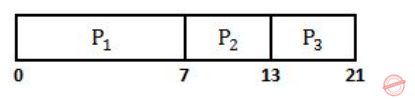BC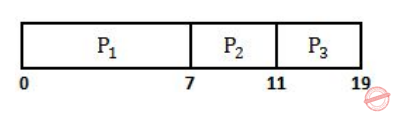DOperating-Systems       Process-Scheduling       UGC NET CS 2018 JUNE Paper-2
Question 54 Explanation:Question 55
Consider a preemptive priority based scheduling algorithm based on dynamically changing priority. Larger priority number implies higher priority. When the process is waiting for CPU in the ready queue (but not yet started execution), its priority changes at a rate a=2. When it starts running, its priority changes at a rate b=1. All the processes are assigned priority value 0 when they enter ready queue. Assume that the following processes want to execute :The time quantum q=1. When two processes want to join ready queue simultaneously, the process which has not executed recently is given priority. The finish time of processes P1, P2, P3 and P4 will respectively be
 A 4, 5, 7 and 8 B 8, 2, 7 and 5 C 2, 5, 7 and 8 D 8, 2, 5 and 7
Operating-Systems       Process-Scheduling       UGC NET CS 2013 Dec-paper-2
Question 55 Explanation:
Given data,Question 56
Preemptive scheduling is the strategy of temporarily suspending a gunning process
 A Before the CPU time slice expires B To allow starving processes to run C When it requests I/O D To avoid collision
Operating-Systems       Process-Scheduling       UGC NET CS 2012 June-Paper2
Question 56 Explanation:
→ Preemptive scheduling is the strategy of temporarily suspending a gunning process before the CPU time slice expires.
→ Preemptive scheduling means the processes is always interrupted by higher priorities.
 Question 57
The only state transition that is initiated by the user process itself is
 A block B dispatch C wakeup D none of the above
Operating-Systems       Process-Scheduling       NIELIT Junior Teachnical Assistant_2016_march
 Question 58
How many states can a process be in ?
 A 3 B 4 C 2 D 5
Operating-Systems       Process-Scheduling       UGC NET CS 2011 June-Paper-2
Question 58 Explanation:
Process states
1. New→ The process is being created.
2. Running→ Instructions are being executed.
3. Waiting→ The process is waiting for some event to occur
4. Ready→ The process is waiting to be assigned to a processor
5. Terminated→ The process has finished execution
 Question 59
A virtual memory based memory management algorithm partially swaps out a process. This is an example of
 A short term scheduling B long term scheduling C medium term scheduling D mutual exclusion
Operating-Systems       Process-Scheduling       UGC NET CS 2013 June-paper-2
Question 59 Explanation:
A virtual memory based memory management algorithm partially swaps out a process. This is an example of medium term scheduling.
Medium-term scheduling:The decision to add to the number of processes that are partially or fully in main memory.
Long-term scheduling:The decision to add to the pool of processes to be executed.
Short-term scheduling:The decision as to which available process will be executed by the processor.
 Question 60
Match the following :A (a)-3, (b)-4, (c)-2, (d)-1 B (a)-4, (b)-3, (c)-2, (d)-1 C (a)-2, (b)-4, (c)-1, (d)-3 D (a)-1, (b)-4, (c)-3, (d)-2
Operating-Systems       Process-Scheduling       UGC NET CS 2010 June-Paper-2
Question 60 Explanation:
Disk Scheduling→ SCAN
Batch Processing→ FIFO
Time Sharing→ Round-robin
Interrupt Processing→ LIFO
 Question 61
_______ is one of preemptive scheduling algorithm.
 A RR B SSN C SSF D Priority based
Operating-Systems       Process-Scheduling       UGC NET CS 2010 June-Paper-2
Question 61 Explanation:
Round Robin(RR) is one of preemptive scheduling algorithm. Priority based scheduling is non preemptive.
 Question 62
Four jobs J1, J2, J3 and J4 are waiting to be run. Their expected run times are 9, 6, 3 and 5 respectively. In order to minimise average response time, the jobs should be run in the order:
 A J1 J2 J3 J4 B J4 J3 J2 J1 C J3 J4 J1 J2 D J3 J4 J2 J1
Operating-Systems       Process-Scheduling       UGC NET CS 2008 Dec-Paper-2
Question 62 Explanation:
Since arrival time is not given assume arrival time of each process as"0" Option (A):Question 63
An example of a non-preemptive scheduling algorithm is :
 A Round Robin B Priority Scheduling C Shortest job first D 2 level scheduling
Operating-Systems       Process-Scheduling       UGC NET CS 2008 Dec-Paper-2
Question 63 Explanation:
→ Round robin and priority scheduling algorithms are preemptive based algorithms.
→ FCFS and SJF is non-preemptive based algorithms but in SJF are supporting another version is SRTF(Shortest remaining time first)
 Question 64
An example of a non-preemptive CPU scheduling algorithm is :
 A Shortest job first scheduling. B Round robin scheduling. C Priority scheduling. D Fair share scheduling.
Operating-Systems       Process-Scheduling       UGC NET CS 2008-june-Paper-2
Question 64 Explanation:
→ Round robin and priority scheduling algorithms are preemptive based algorithms.
→ FCFS and SJF is non-preemptive based algorithms but in SJF are supporting another version is SRTF(Shortest remaining time first)
 Question 65
Consider the following four processes with the arrival time and length of CPU burst given in milliseconds :The average waiting time for preemptive SJF scheduling algorithm is __________.
 A 6.5 B 7.5 C 6.75 D 7.75
Operating-Systems       Process-Scheduling       UGC NET CS 2017 Nov- paper-3
Question 65 Explanation:Question 66
Some of the criteria for calculation of priority of a process are:
a. Processor utilization by an individual process.
b. Weight assigned to a user or group of users.
c. Processor utilization by a user or group of processes In fair share scheduler, priority is calculated based on:
 A only (a) and (b) B only (a) and (c) C (a), (b) and (c) D only (b) and (C)
Operating-Systems       Process-Scheduling       UGC NET CS 2017 Jan- paper-3
Question 66 Explanation:
Fair-share scheduling is a scheduling algorithm for computer operating systems in which the CPU usage is equally distributed among system users or groups, as opposed to equal distribution among processes.
 Question 67
Consider three intensive processes, which requires 10,20 and 30 units of time and arrive at times 0,2 and 6 respectively. how many context switches are needed if the operating system implements a shortest remaining time first scheduling algorithm? Do not count the context switches at time zero ad at the end
 A 4 B 2 C 3 D 1
Operating-Systems       Process-Scheduling       UGC NET June-2019 CS Paper-2
Question 67 Explanation:Total no.of context switches is 2.
 Question 68
Which of the following statements is not true for Multi Level Feedback Queue processor scheduling algorithm?
 A Queues have different priorities B Each queue may have different scheduling algorithm C Processes are permanently assigned to a queue D This algorithm can be configured to match a specific system under design
Operating-Systems       Process-Scheduling       UGC NET CS 2015 June Paper-3
Question 68 Explanation:
MultiLevel Feedback queue:
● There are more than one queues and each queue have assigned a different priority.
● Since there are more than one queue, therefore more than one scheduling algorithm is applied.
● Job starts in the highest priority queue.
● If time slice expires, drop the job by one level and if time slice does not expire push the job up by one level.
● This algorithm can be configured to match a specific system under design
 Question 69

Match the following .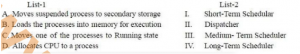A A-III, B-IV, C-I, D-II B A-II, B-I, C-IV, D-III C A-III, B-I, C-II, D-IV D A-II, B-IV, C-I, D-III
Operating-Systems       Process-Scheduling       CIL Part - B
Question 69 Explanation:

Moves suspended process to secondary storage → Medium Term Scheduler

Loades the process into memory for execution → Long Term Scheduler

Moves one of the process to running time → Short Term Scheduler

Allocate CPU to a process → Dispatcher
 Question 70
Given the Burst Time (BT) of 4 processes P1, P2, P3 and P4 as BT (t1,t2,t3,t4)=(4,8,6,7), smoothening factor()=0.5, and T1=10, what will be the burst time of process P5 for shortest job first scheduling, using the technique of exponential averaging ?
 A 6.875 unit time B 6.25 unit time C 5.785 unit time D 5.5 unit time
Operating-Systems       Process-Scheduling       CIL Part - B
Question 70 Explanation:Question 71

Considering the process details given in the following table, what will be the completion order of the processes under the policies FCFS and RR (with CPU quantum of 2 time units)?A FCFS: P1, P2, P3 and RR: P1, P2, P3 B FCFS: P3, P2, P1 and RR: P3, P1, P2 C FCFS: P3, P2, P1 and RR: P3, P2, P1 D FCFS: P1, P3, P2 and RR: P1, P2, P3
Operating-Systems       Process-Scheduling       CIL Part - B
Question 71 Explanation: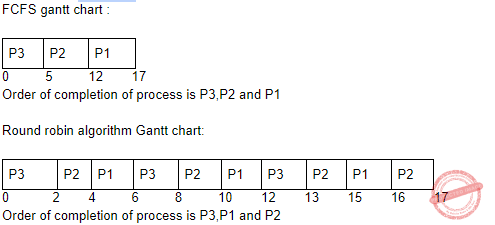Question 72

Consider the process details given in the following table.A new pre-emptive scheduling algorithm is proposed, i.e., the Longest Remaining Time Next (LRTN), wherein ties are broken by giving priority to the process with the highest priority
.
Calculate the following.

i. Turnaround time for Process P2

ii. Response time for Process P3
 A (i)20, (ii)6 B (i)19, (ii)3 C (i)19, (ii)8 D (i)20, (ii)7
Operating-Systems       Process-Scheduling       CIL Part - B
Question 72 Explanation:Question 73
Which of the following algorithms defines time quantum?
 A Shortest job scheduling algorithm B Round robin scheduling algorithm C Priority scheduling algorithm D Multilevel queue scheduling algorithm
Operating-Systems       Process-Scheduling       ISRO CS 2020       Video-Explanation
Question 73 Explanation:
Round Robin uses time quantum to fairly allocate CPU for each process in the system.
 Question 74
Dispatch latency is defined as
 A The speed of dispatching a process from running to the ready state B The time of dispatching a process from running to ready state and keeping the CPU idle C The time to stop one process and start running another one D None of these
Operating-Systems       Process-Scheduling       ISRO CS 2020       Video-Explanation
Question 74 Explanation:
The time it takes for the dispatcher to stop one process and start another is known as the dispatch latency
 Question 75
Three CPU-bound tasks with execution times of 15, 12 and 5 time units respectively arrive at times , t and 8, respectively. If the operating system implements a shortest remaining time first scheduling algorithm, what should be the value of t to have 4 context switches?
Ignore the context switches at time 0 and at the end.
 A 0 < t < 3 B t = 0 C t <= 3 D 3 < t < 8
Operating-Systems       Process-Scheduling       ISRO CS 2020       Video-Explanation
Question 75 Explanation:
In the question it asked to find the arrival time (t) of process (P2), such that the number of context switches is exactly 4 using SRTF scheduling algorithm. It can be simply verified by taking t=1 and t=2, which causes 4 context switches. So, 0< t < 3. At, t=0 and and t >=3, no of context switches are not exactly 4.
 Question 76
The above table depicts the CPU burst times required for the processes P1, P2, P3 and P4 which are in ready queue at a given moment. What is the average waiting time resulted with priority scheduling of CPU giving highest priority to level-1?
 Process ID Priority level CPU Burst time P1 2 10 P2 3 8 P3 1 12 P4 4 5
 A 16 B 24.75 C 18 D 25
Operating-Systems       Process-Scheduling       APPSC-2016-DL-CS
Question 76 Explanation:Question 77

In processor management, round-robin method essentially uses the pre-emptive version of

 A FILO B FIFO C SJF D longest time first
Operating-Systems       Process-Scheduling       APPSC-2016-DL-CA
Question 77 Explanation:
Round Robin method uses FIFO, i.e., First In First Out.
 Question 78

Consider the following table of arrival time and burst time for three processes P0,P1,P2The preemptive shortest job first scheduling algorithm is used scheduling is carried out only at arrival or completion of process. The average waiting time for three process is____.

 A 7 Seconds B 4 seconds C 6 seconds D 5 seconds
Operating-Systems       Process-Scheduling       CIL 2020
Question 78 Explanation:∴ Avg. WT = 4+0+11/3 = 5
 Question 79

Which scheduling algorithm gives a minimum average waiting time?

 A RR B Priority C SJF D FCFS
Operating-Systems       Process-Scheduling       CIL 2020
Question 79 Explanation:
Shortest Job First (SJF) is an algorithm in which the process having the smallest execution time is chosen for the next execution. This scheduling method can be preemptive or non-preemptive. It significantly reduces the average waiting time for other processes awaiting execution.
 Question 80

In which Disk scheduling algorithm starvation is possible?

 A FCFS B SCAN C Shortest Seek Time First (SSTF) D C-SCAN
Operating-Systems       Process-Scheduling       APPSC-2012-DL CA
Question 80 Explanation:
In SSTF the request with largest seek time may suffer if a request of low seek time comes.
 Question 81

Which scheduling algorithm from the following is allows processes to move from one queue (different classified queues based on scheduling parameters) to another?

 A Multilevel Queue Scheduling B Priority Based Scheduling C Round Robin Scheduling D Multilevel Feedback Queue Scheduling
Operating-Systems       Process-Scheduling       APPSC-2012-DL CA
Question 81 Explanation:
Multilevel feedback queue scheduling solves the problem of starvation of low priority processes in multilevel scheduling and in this the processes can move from one queue to another.
 Question 82
In a Round Robin CPU scheduling, as the time quantum is increased, the average turn-around time
 A Increases B Decreases C Remains constant D Varies irregularly
Operating-Systems       Process-Scheduling       TNPSC-2012-Polytechnic-CS
Question 82 Explanation:
In round robin scheduling algorithm time quantum is nowhere related to turn around time.
 Question 83
Multiprogramming means
 A Executing more than one program at a time B Ability to accommodate multiple tasks in main memory C Presence of multiple processors in one system D All the above
Operating-Systems       Process-Scheduling       APPSC-2012-DL-CS
Question 83 Explanation:
Multiprogramming means the ability to put aor place more than one process in the main memory.
 Question 84
Consider a uniprocessor system where new processes arrive at an average of five processes per minute and each process needs an average of 6 seconds of service time. What will be the CPU utilization ?
 A 80 % B 50 % C 60 % D 30 %
Operating-Systems       Process-Scheduling       UGC NET CS 2014 June-paper-3
Question 84 Explanation:
5 processes arrives in 60seconds.
Service time for 1 process--------> 6sec
Service time for 5 processes------> 5*6 sec
= 30 seconds
CPU utilization = (30/60)*100
= 50%
 Question 85

In the following process state transition diagram for a uniprocessor system, assume that there are always some processes in the ready state. Now, consider the following statements.I. If a process makes a transition D, it would result in another process making transition A immediately

II. A process P2 in blocked state can make transition E while another process PI is in running state

III. The OS uses preemptive scheduling

IV. The OS uses non-preemptive scheduling

Which of the above statements are TRUE?
 A I and II B I and III C II and III D II and IV
Operating-Systems       Process-Scheduling       HCU PHD CS MAY 2019
Question 85 Explanation:
S1 is false because If a process makes a transition D ,then it would result in another process making transition B immediately and not A.
S2 is true.
S3 is true due to the transition C, because transition C states that if a process is in running state it can be pulled out to a ready state when some high priority process comes in the ready state.
S4 is false ,since S3 is true.
 Question 86
Jobs keep arriving at a processor. A job can have an associated time length as well as a priority tag. New jobs may arrive while some earlier jobs are running. Some jobs may keep running indefinitely. A starvation free job-scheduling policy guarantees that no job waits indefinitely for service. Which of the following job-scheduling policies is starvation free?
 A Round-robin B Shortest job first C Priority queuing D Latest job first E None of the others
Operating-Systems       Process-Scheduling       TIFR PHD CS & SS 2020
There are 86 questions to complete.